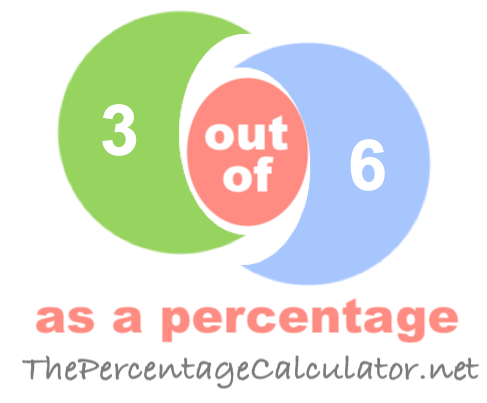What is 3 out of 6 as a percentage?When you ask "What is 3 out of 6?" you want to know what percent 3 is out of 6.

Here are step-by-step instructions showing you how we calculated 3 out of 6 as a percentage:

The first step is to divide 3 by 6 to get the answer in decimal form:

3 / 6 = 0.5000

Then, we multiplied the answer from the first step by one hundred to get the answer as a percentage:

0.5000 * 100 = 50.00%

We can prove that the answer is correct by taking 50.00 percent of 6 to get 3:

(6 x 50.00)/100 = 3

Note that our calculator rounds the answers up to two decimals if necessary. Once again, the answer is as follows:

50.00%

Out of as a Percentage Calculator
To solve another problem, please submit it below:

out of

What is 3 out of 7 as a percentage?
The solution to "What is 3 out of 6 as a percentage?" is not the only answer we have. Go here for the next solution on our list.